Courses

# Variance Analysis - Cost Accounting Techniques, Cost Accounting B Com Notes | EduRev

## B Com : Variance Analysis - Cost Accounting Techniques, Cost Accounting B Com Notes | EduRev

The document Variance Analysis - Cost Accounting Techniques, Cost Accounting B Com Notes | EduRev is a part of the B Com Course Cost Accounting.
All you need of B Com at this link: B Com

Variance Analysis
The primary object of standard costing is to reveal the difference between actual cost and standard cost. A ‘variance’ in standard costing refers to the divergence of actual cost from standard cost. Variances of different cost items provide the key to cost control. They indicate whether and to what extent standards set have been achieved. This enables management to correct adverse tendencies. After standard costs have been established, the next step is to ascertain the actual cost under each element and compare them with the standard cost. The difference between these two is termed as cost variance. Cost variance is the difference between a standard cost and the comparable actual cost incurred during a given period.
The Chartered Institute of Management Accountants London, defines variance as “the difference between planned, budgeted, or standard cost and actual cost; and similarly for revenue”.
Variance analysis can be defined as “the analysis of performance by means of variances”. It is the process of computing the amount of and isolating the cause of variances between actual costs and standard costs.

Variance analysis involves:
(a) Computation of individual variances, and
(b) Determination of the cause(s) of each variance.
Actual cost which is higher than the standard costs would be a sign of inefficiency and the difference would be termed as unfavourable or adverse. A variance that reduces profit is adverse or unfavourable. A variance that increases profit is favourable. Variance are computed under each element of cost for which standards have been established. Each variance is analysed to ascertain the causes so that the management can exercise proper control. The cause is affixed to the variance, for example, materials price variance will show that the variance arose due to change in the price of materials. Some of the variance are controllable while others are not. The purpose of such classification is that proper emphasis can be placed on the controllable variance. This follows the principle of management by exception.
Variances occurring in a period may be compared with variances on the same account expressed as a percentage of the standard costs and compared with the percentage for the previous month. Comparison may be made between the standard and actual or between basic standard and current standard.
As already stated, the origin and causes of the variances need to be traced by analysing the total variances into their components parts in order to determine and isolate the causes giving rise to each variance.
Equal emphasis should be laid on favourable and unfavourable variances. An unfavourable variance points out the inefficiency in use or waste of materials, labour, and resources. A favourable variance may be due to improvement in efficiency or production of substandard products or an incorrect standard. An unfavourable variance may be off-set by a favourable variance; hence the need for analysis and appropriate action.
A detailed probe into the variances, particularly the controllable variance, helps the management to ascertain:
(a) the amount of variance;
(b) its occurrence;
(c) the factors responsible for it;
(d) the executive responsible for the variance;
(e) corrective action which should be taken to obviate or reduce the variance.

Favourable and Unfavourable Variance: If the actual cost is less than standard cost, the difference is known as a favourable variance, credit variance or positive variance denoted by (F) or Cr. - it increases the profit. on the other hand, if actual cost exceeds, standard costs, the divergence is known as an unfavourable variance, debit variance, negative variance or adverse variance denoted by (A) or Dr. - it reduces the profit.

Controllable and Uncontrollable Variance: When the variance with respect to any cost item reflects the degree of efficiency of an individual or department, i.e., a particular individual or departmental head is responsible for the variance, the variance is known as a controllable variance. Obviously, such a variance is amenable to control by suitable action. An uncontrollable variance is one which is not amenable to control by individual or departmental action. Such a variance is caused by external factors like change in market conditions, fluctuations in demand and supply, etc. No particular individual within the organisation can be held responsible for it.
When variances are reported, attention of the management is particularly drawn towards controllable variances. If a variance has been caused by multiple factors, the part of cost variance relevant to each factor should be determined.
There are certain variances which may arise under material, labour or overhead due to change in the basic condition on which the standards are established.

Revision Variance: This is amount by which a budget is revised but which is not incorporated in the standard cost rate as a matter of policy. The standard costs may be affected by wage rate changes after wage accords, fiscal policy etc. The standard costs are not disturbed to account for these uncontrollable factors and to avoid the amount of labour and cost involved in revision, the basic standard costs are allowed to stand. It is essential to isolate the variance arising out of non-revision in order to analyse the other variances correctly.

Method Variance: It is the difference between the standard cost of the product manufactured or operation performed by the normal methods and the cost of operation by alternative method. Standards usually take into account the best method applicable, and any deviation will result in an unfavourable variance. Hence such deviations should be as few as possible.
Variance analysis usually proceeds after amending the standards according to the revision variance and the methods of variance.

Illustration 1
Standard cost of a product in a factory is predetermined as follows:

 Rs. Material (5 units @ Rs. 4 each) 20 Labour (20 hours @ Rs. 1.50 per hour) 30 Overhead expenses 10 Total 60

During a period, 8,000 units were produced whose actual cost was as follows:

 Rs. Material (40,500 units @ Rs. 5 each) 2,02,500 Labour (1,50,000 hours @ Rs.1.60 each) 2,40,000 Overhead expenses 90,000 Total 5,32,500

Prepare a statement showing standard cost, actual cost and variances.

Solution:
Statement of Standard Cost, Actual Cost, and Variances

 Particulars Standard cost (Rs) Actual cost (Rs) Variance (Rs) Material 1,60,000 2,02,500 42,500 (A) Labour 2,40,000 2,40,000 — Overhead expenses 80,000 90,000 10,000 (A) Total 4,80,000 5,32,500 52,500 (A)

The above statement shows the variance in respect of each element of cost. Each such variance can be further analysed. Before making such analysis it is necessary to recognise the two broad process in cost accumulation. The cost is first incurred and then charged to production. For example, materials are purchased first (normally) and then issued for production and wages are incurred first and then charged to production on the basis of time spent on production. Thus, there are two stages in cost accumulation, namely, (i) the incurring stage, (ii) the recovery stage. The recognition of these two stages is essential because variances arise both at the incurring and recovery stages. Analysis involves identifying and quantifying the variances at both these stages.
Before we proceed to analyse the variances, the following essential points should be noted regard to the utility of the variance analysis:

1. Variances should not be automatically applied for control purposes. They are just indicators of where the reason for higher cost exists. It is upto the controlling authority to judge whether the higher costs are well justified. The actual cost may be higher due to factors absolutely out of the control of the responsible authority and perhaps the responsible authority had contributed in preventing the actual cost from escalating too high. In such a situation applying controls implicit on the basis of variances disclosed will lead to demoralisation of staff.
2. While comparing the actual costs with the standards, the level of activity should be checked up for comparability. If standards have been evolved for a budgeted level of activity and if the actual level is different, a simple comparison of actuals with budgets would be erroneous. The standards should be revised in accordance with the actual level of activity attained. But, in doing so care should be taken to distinguish between fixed costs and variable costs. The difference between the original standard and revised standard is known as “Revision Variance”.
3. While working out the variance in respect of fixed costs (particularly fixed overheads), it should be kept in mind that what is charged to cost is not the actual cost but an amount based on predetermined recovery rates multiplied by the output which may be expressed in standard hours.

Two-Way Analysis of Variances
Each variance has to be analysed as (i) incurring variance, and (ii) recovery variance. Also, broadly, the causes leading to a variance may be either efficiency or inefficiency in the use of resources or change in the price paid for the resources. Accordingly, we have the following analysis:

 (i) Material cost variance - Material price variance- Material usage variance (ii) Labour cost variance - Labour rate variance- Labour time variance (iii) Overheads cost variance - Overhead expenditure variance- Overhead volume variance

As each element of cost is analysed into two broad groups. It is known as “Two- way Analysis”.

TYPES OF VARIANCES
There is a need of knowing types of variances before measuring the variances. Generally, the variances are classified on the following basis.

A. On the basis of Elements of Cost.

1. Material Cost Variance.
2. Labour Cost Variance.
3. Overhead Variance.

B. On the basis of Controllability

1. Controllable Variance.
2. Uncontrollable Variance.

C. On the basis of Impact

1. Favorable Variance.
2. Unfavorable Variance

D. On the basis of Nature

1. Basic Variance.
2. Sub-variance.

A brief explanation of the above mentioned variances are presented below
1. Material Cost Variance
It is the difference between actual cost of materials used and the standard cost for the actual output.
2. Labour Cost Variance
It is the difference between the actual direct wages paid and the direct labour cost allowed for the actual output to be achieved.
3. Overhead Variance
Overhead variance is the difference between the standard cost of overhead allowed for actual output (in terms of production units or labour hours) and the actual overhead cost incurred.
4. Controllable Variance
A variance is controllable whenever an individual or a department or section or division may be held responsible for that variance.
According to ICMA, London,
5. Uncontrollable Variance
External factors are responsible for uncontrollable variances. The management has no power or is unable to control the external factors. Variances for which a particular person or a specific department or section or division cannot be held responsible are known as uncontrollable variances.
6. Favourable Variances
Whenever the actual costs are lower than the standard costs at per-determined level of activity, such variances termed as favorable variances. The management is concentrating to get actual results at costs lower than the standard costs. It shows the efficiency of business operation.
7. Unfavorable Variances
Whenever the actual costs are more than the standard costs at predetermined level of activity, such variances termed as unfavorable variances. These variances indicate the inefficiency of business operation and need deeper analysis of these variances.
8. Basic Variances
Basic variances are those variances which arise on account of monetary rates (i.e. price of raw materials or labour rate) and also on account of non-monetary factors (such as physical units in quantity or time). Basic variances due to monetary factors are material price variance, labour rate variance and expenditure variance. Similarly, basic variance due to non-monetary factors are material quantity variance, labour efficiency variance and volume variance.
9. Sub Variance
Basic variances arising due to non-monetary factors are further analyzed and classified into sub-variances taking into account the factors responsible for them. Such sub variances are material usage variance and material mix variance of material quantity variance.
Likewise, labour efficiency variance is subdivided into labour mix variance and labour yield variance. At the same time, variable overhead variance is sub-divided into variable overhead efficiency variance and variable overhead expenditure variance.

ADVANTAGES OF VARIANCE ANALYSIS
The following are the merits of variance analysis.
1. The reasons for the overall variances can be easily find out for taking remedial action.
2. The sub-division of variance analysis discloses the relationship prevailing between different variances.
3. It is highly useful for fixing responsibility of an individual or department or section for each variance separately.
4. It highlights all inefficient performances and the extent of inefficiency.
5. It is used for cost control.
6. The top management can follow the principle of management by exception. Only unfavorable variances are reporting to management.
7. Sometimes, the variances can be classified as controllable and uncontrollable variances. In this case, controllable variances are taken into consideration for further action.
8. Profit planning work can be properly carried on by the top management.
9. The results of managerial action can be a cost reduction.
10. It creates cost consciousness in the minds of the every employee of business organization.

Variance Analysis Example:
(A Comprehensive illustration of all variances)

Example: The Springmint Company, a manufacturer of chewing gum, uses a standard cost system. Standard product and cost specifications for 1,000 lbs. of chewing gum are as follows:
Answer: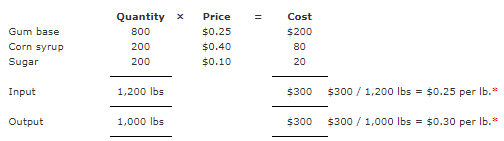Example: The production of 1,000 lbs. of chewing gum required 1,200 lbs of raw materials. Hence the yield is 1,000 lbs / 1,200lbs. or 5/6 of input. Materials records indicate.
Answer: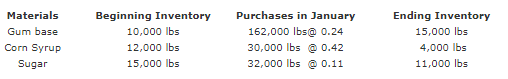Example: To convert 1,200 lbs. of raw materials into 1,000 lbs of finished product required 20 hours at \$6.00 per hour or \$0.12 per lbs. of finished product. Actual direct labor hours and cost for January are 3,800 hours at \$23,104. Factory overhead is applied on a direct labor hour basis at a rate of \$5 per hour (\$3 fixed , \$2 variable), or \$ 0.1 per lb. of finished product. Normal overhead is \$20,000 with 4,000 direct labor hours. Actual overhead for the month is \$22,000, Actual finished production for January is 200,000 lbs.
Answer: The standard cost per pound of finished chewing gum is: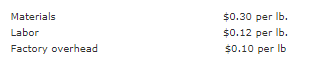Calculation of Materials Variance:

The materials variances for January consists of  price variance, mix variance,  yield variance, and quantity variance.

Materials Price Variance:

Example: The company calculates the materials price variance using the procedure explained on "direct materials price variance" page and recognizes variances when materials are purchased.
Answer:The company calculates the materials price variance using the procedure explained on "direct materials price variance" page and recognizes variances when materials are purchased.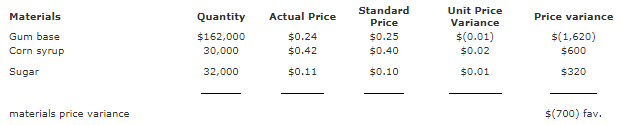Example:The materials mix variance results from combining materials in a ratio different from the standard materials specifications. It is computed as follows:
Answer: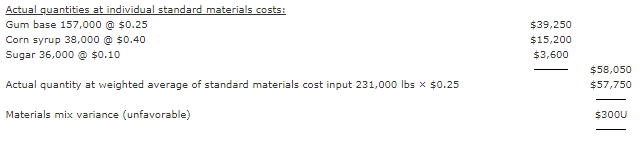Materials Yield Variance:

Material yield variance is computed as follows:
Example: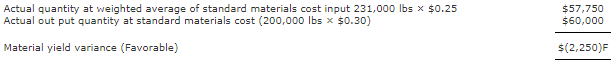F = Favorable
U = Unfavorable
The yield variance occurred because the actual production of 200,000 lbs. exceeded the expected output of 192,500 lbs. (5/6 of 231,000) by 7,500 lbs. The yield difference multiplied by the standard weighted materials cost of \$0.30 per output pound equals the favorable yield variance of \$2,250.
The materials quantity variance can be calculated for each item as follows, using the procedure explained on direct materials quantity variance page.

Answer: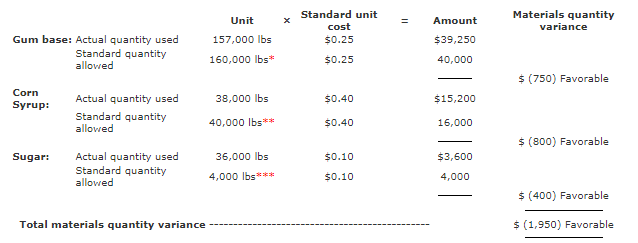• An output of 200,000 lbs. should require and output of 240,000 lbs., with a standard yield of 1,000 lbs. output for each 1,200 lbs input. Then the 240,000 lbs. × (800 lbs. / 1,200 lbs ) gum base portion of the formula = 160,000 lbs.
• The 240,000 lbs. × (200lbs. / 1,200 lbs.) corn syrup portion of the formula = 40,000 lbs
• The 240,000 lbs. × (200lbs. / 1,200 lbs.) sugar portion of the formula = 40,000 lbs.

Example: The total materials quantity variance can also be determined by comparing actual quantities at standard prices, \$58,050 (\$39250 + \$15,200 + \$3,600), to actual output quantity at standard materials cost, \$60,000 (200,000 lbs × \$0.30) for a total favorable variance of \$1,950. The mix and yield variances separate the materials quantity variance into two parts:
Answer: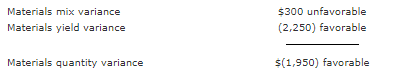Example: The influence of individual raw materials on the total materials mix variance can be calculated in the following manner:
Answer: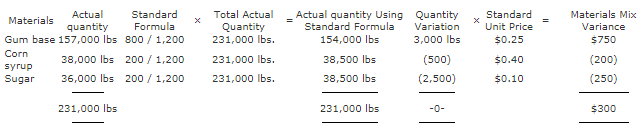The expected output of 192,500 lbs. of chewing gum should require 3,850 standard labor hours (20 hours per thousand pounds of chewing gum produced). Similarly, the actual out put of 200,000 lbs. of chewing gum should require 4,000 standard labor hours.
The labor variances are labor rate variance, labor efficiency variance and labor yield variance.

Offer running on EduRev: Apply code STAYHOME200 to get INR 200 off on our premium plan EduRev Infinity!

## Cost Accounting

131 videos|139 docs

,

,

,

,

,

,

,

,

,

,

,

,

,

,

,

,

,

,

,

,

,

,

,

,

;